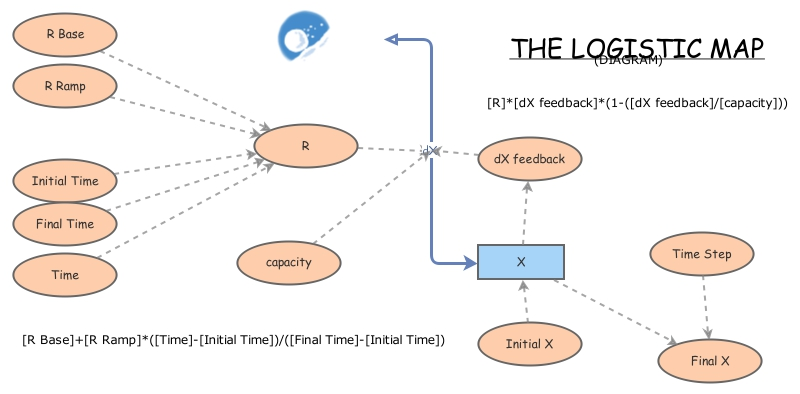The Logistic MapThe Logistic Map is a polynomial mapping (equivalently, recurrence relation) of degree 2, often cited as an archetypal example of how complex, chaotic behaviour can arise from very simple non-linear dynamical equations. The map was popularized in a seminal 1976 paper by the biologist Robert May, in part as a discrete-time demographic model analogous to the logistic equation first created by Pierre François Verhulst.

Mathematically, the logistic map is written$(1)\qquad x_{n+1} = r x_n (1-x_n)$

where:$x_n$ is a number between zero and one, and represents the ratio of existing population to the maximum possible population at year n, and hence xrepresents the initial ratio of population to max. population (at year 0)
r is a positive number, and represents a combined rate for reproduction and starvation.
To generate a bifurcation diagram, set 'r base' to 2 and 'r ramp' to 1
To demonstrate sensitivity to initial conditions, try two runs with 'r base' set to 3 and 'Initial X' of 0.5 and 0.501, then look at first ~20 time steps